## Mathematics Paper 2 Questions and Answers - Mang'u Mock 2020 Exam

MATHEMATICS
PAPER 2
TIME: 2 HOURS.

Instructions To Candidates

• This paper contains two sections: section I and section II
• Marks may be given for correct working even if the answer is wrong.
• Non programmable silent electronic calculators and KNEC mathematical tables may be used, except where stated otherwise.

SECTION A (50 MARKS)
Answer all questions in the spaces provided

1. Evaluate (3mks)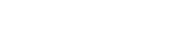2. The point (9, 4) is the image of the point (1,4) under a shear with x-axis invariant. Find the matrix of the shear.(2mks)
3. In vectors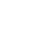,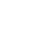, , find x and y given that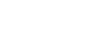(3mks)
4. Triangle ABC is such that AB =10cm, angle BAC=60° and angle ABC = 30°
1. construct triangle ABC and construct the locus of P such that the area of triangle APB is 20cm2 (2mks)
2. Indicate the locus of Q such that Q is equidistant from CA and CB (2mks)
5. In the figure below, VABCD is a right pyramid on a rectangular base. Point O is vertically below the vertex V. AB=24cm, BC=10cm and CV=26cm Calculate the angle between the edge CV and the base ABCD (2mks)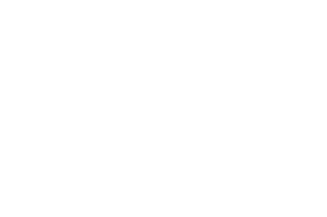6. The escribed circle of triangle ABC touches BC at P, AB produced at Q, and AC produced at R. If AQ=10cm, AR=10cm and AO = 12cm, find the length of OQ and shaded area(4mks)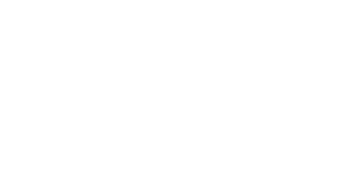7. Make K the subject at the formular(3mks)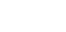8. Solve for x if logx16 + logx32 = 18 (3mks)
9. Simplify without using table leaving your answer in the form a+ b√c
1 + cos 30° sin 45°
1 - sin 60° cos 45º
10. Y varies inversely as the square of x. The difference between the values of y when x=6 and when x=10 is 6. Find x if y=4 (3mks)
11. A ship sails due East from an island (48°N, 42°E) to another island B. The average speed of the ship is 30 knots and it takes 22 hours to reach island B. Find position of island B. (3mks)
12. Solve the equation 6sin?0 - cose - 5 = 0, where 360° ≥ θ ≥ 0º (3mks)
13. Calculate the standard deviation of the following data. (3mks)
 No of goals 0 1 2 3 4 5 6 No. of matches 11 2 5 8 3 2 1
14. The figure below shows a quadrilateral ABCD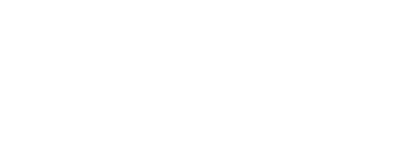Calculate the length of BD and AB (4mks)
15. Given that x=6.4 and y=5, find the correct to 1 decimal place, the percentage
error in   xy   (3mks)
x+y
16. The angle of elevation of the top of a flag post from a point A on a level ground is 23°. The angle of elevation of the top of the flag from another point B nearer the flag post and 100m from A is 40°. A, B and the bottom of the flag are collinear
Calculate
1. the distance from the point B to the top of the flag post(2mks)
2. the height of the flag post (2mks)

SECTION B (50 MARKS)
Answer all questions in the spaces provided

1. A community water tank is in the shape of a cuboid of base 6m by 5m and height of 4m. A feeder pipe of diameter 14cm supplies water to this tank at a flow of 40cm/s.
Calculate
1.
1. the capacity of the tank is litres (2mks)
2. the amount of water, in litres, delivered to this tank in one hour (3mks)
3. the time taken for the tank to fill (2mks)
2. The community consumes a full tank a day, with each family consuming an average of 150 litres per day. If each family pays a uniform rate of sh.350 per month, find the total amount of money due monthly (3mks)
2. In driving to work, Jane has to pass through three sets of traffic lights. The probability that she will have to sop at any of the lights is 3/5.
1. Draw a tree diagram to represent the above information(2mks)
2. Using the diagram, determine the probability that on any one journey, she will have to stop at
1. all the three sets(2mks)
2. only one of the sets (2mks)
3. only two of the sets (2mks)
4. none of the sets(2mks)
3.
1. On the grid provided, draw the graph of the functions y = ½x2 - x+3 for 0 ≤ x ≤  6
2. Calculate the mid-ordinates for 5 strips between x=1 and x=6 and hence use the mid-ordinate rule to approximate the area under the curve between X=1, x=6 and the x-axis (3mks)
3. Assuming that the area determined by integration to be the actual area. Calculate the percentage error in using the mid-ordinate rule. (4mks)
4.
1. Sketch the curve of y=(x-3) (2x2 - 3x + 1) (5mks)
2. Given that a cone whose base radius and perpendicular height are rcm and hcm respectively. (1cm)
Determine
1. the radius r in terms of h if the slant height is 10cm(1mk)
2. value of perpendicular height, H, which the volume of the cone is maximum and hence find the maximum possible volume (4mks)
5. A carpenter takes 4 hours to make a stool and 6 hours to make a chair. It takes the carpenter at least 144 hours to make x stools and y chairs. The labour cost of making a stool is ksh.100 and that of a chair is ksh.200. The total labour cost should not exceed ksh.4,800. The carpenter must make at least 16 stools and more than 10 chairs.
1. Write down inequalities to represent the above information(3mks)
2. Draw the inequalities in (a) above on the grid provided(4mks)
3. The carpenter makes a profit of ksh.40 on a stool and ksh100 on a chair. Use the graph to determine the maximum profit the carpenter can make. (3mks)

MARKING SCHEME

SECTION A

1.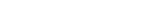(x4 - x3 + x2 -2x)2
=[16-8 +4-4]- [16+8+4-4]
= 16
2.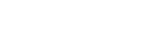1 + 4k = 9
4k=8
k= 2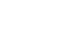3.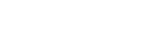x = 16 = x=4
-3x - y = 11 =y=-23
4.
1.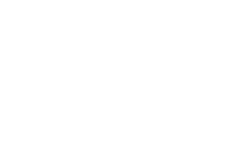2. Locus θ labeled
Bisector of AC and BC
5. Diagonal = √242 - 102 = 26
Cost = 13/26 -0 = 600
6. OQ=√122 - 102
= √44
= 6.633
Sinθ = 10/12
θ = 56.44
Area Δ AOQ = ½ x 6.633 x 10 = 33.165cm2
Area sector OQP = 56.44 x 22/7 x 6.635
360
= 21.68cm2
= 22.97cm2

7. R2 = k2 + a2/Hg
K2 = hgR2 - a2
K =±√hgR2 - a2

8. 4logx2 + 5logx2 = 18
9 logx2= 18
Logx2 = 2
x2 = 2
x=√2 = 1.4142

9.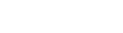=4+√6   4
4        4-√6
4+√6(4+√6) = 16+8√6 + 6
4-√6 (4+√6)          10
=11 + 4√6
5

10. Y =k
x2
k   -   k  = 6
36     100
64k =6
3600
K-337.5
4 = 337.5
X2
X = √337.5
4
= 9.186

11. D = SXT
22 x 30 = 660nm
60θ = 660
θ = 110
B (48°N, 53°E)

12. 6(1-сos2 θ) - cos θ -5 = 0
6 - 6cos2θ - cos θ -5 =0
6cos2 θ + cosθ -1 = 0
6cos2θ +3 cosθ - 2cos θ-1 = 0
3 cosθ(2cosθ+1)-1(2cosθ+1) = 0
(3cosθ-1) (2cosθ+1)=0
cosθ =1/3 or cosθ = -1/2
θ= 150°, 210°, 70.53°, 289.47°

13.
 X F Fx d=x-m d2f 0 11 0 -2 0 1 2 2 -1 1 2 5 10 0 0 3 8 24 1 3 4 3 12 2 16 5 2 10 3 45 6 1 6 4 96 32 64 161
Mean = ΣFx
fx
= 64/32
=2
s.d=√Σd2f
Σf
=√5.031 = 2.243

14. BD2 - 152 +102 -2(15)(10) cos40
BD2 = 95.19
BD = 9.756
12 9.756
sinB    sin50
sinB = 12 sin50
9.756
B = 70.43; D = 59.57
AB    = 9.756
Sins9.57    sin50
AB = 10.98cm

15. Vw =6.4(5) = 2.8070
6.4+5
Vmax = 6.45(5.5) = 3.2696
4.5+6.35
Vmin = 6.35(4.5) = 2.3912
6.45+5.5
A.E = 3.2696 - 2.3912 = 0.4392
2
%E=0.4392 x 100 - 15.6%
2.8070
16.

1.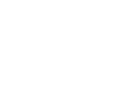tan40 = h/x
tan23= h/100+x
xtan40=(100+x) tan23
0.8391x -0.4245 = 42.45
x =102.38m
PB = 102.382 +85.912 =133.65m
or cos40-102.35/PB = PB = 133.65m

2. Height = xtan40 = 102.38 x tan40 = 85.91m

SECTION B

1.
1.
1. Capacity = 6 x 5 x 4 x 1000
= 120000L
2. Amount = (22/7 x 72 x 40 x 60 x 60)/100
=22176Litres
3. Time = 120000
22176
=5.411 hours

2. No. of families = 120000/150 = 800
Amount = 800 x 350
= 280,000
2.
1.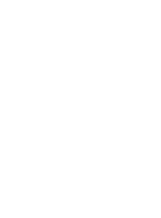2.
1. P(3S) = 3/5 x 3/5 x 3/5 =9/125
2. P (Is only)
3/5 x 2/5 x 2/5 + 2/5 x 3/5 x 2/5 + 2/5 x 2/5 x 3/
=36/125
3. P (2s only)
3/5 x 3/5 x 2/5 + 3/5 x 2/5 x 3/5 + 2/5 x 3/5 x 3/
=54/125
4. P (none) = 2/5 x 2/5 x 2/=8/125
1.
 x 0 1 2 3 4 5 6 y 3 2.5 3 4.5 7 10.5 15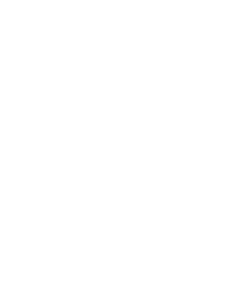2. y1=2.6, y2=3.6, y3=5.6, y4 =8.6, y5=12.6
Area = 1 [2.6+3.6+5.6_8.6+12.6]
= 33 sq. units

3.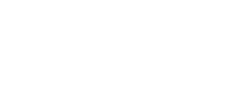= 36 - 22/3
= 331/3
% error = 1/3    x 100
100
= 1.01%
1. x-intercept = when y=o
(x-3)(2x2 - 3x + 1)=0
X=3 or 2x - 2x -x+1=0
(2x - 1)(x-1)=0
X= ½ or 1
(3,0), (½,0), (1, 0) y-intercept = when x=0
y=-3 (0, -3)
St. pts=y=2x3 - 9x2 + 10x -3
dy/dx = 6x2 - 18x + 10
6x2 - 18x + 10 = 0
X = 2.264 or 0.7362
St pts. (0.7362, 0.2821)
And
(2.264, -3.282)
Nature of st. pts
d2y/dx2 = 12x - 18
when x=0.7362
d2y=-ve
dx2
(0.7362, 0.2821) = Max st. pt
When x = 2.264
d2y=+ve
dx2
(2.264, -3.282) = Min st. pt.

2.
1. r2 = 100-h2 = r = √100 - h2
2. V=1/3π(100 - h2)h = v= 100πh1/3
3
dv = 100π - πr2 = dv=0
dh      3                dh
h= √100/3 - 5.773
3.
1. 4x + 6y ≥ 144
100x + 200y ≤ 4800
X ≥ 16 and y > 10
2.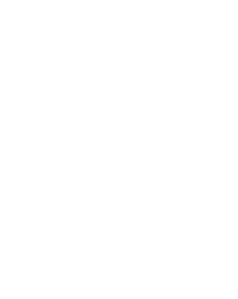3. Max point (16,16)
Objective function: z=40x + 100y
Profit = 40(16) + 100 (16)
=sh 2240

• ✔ To read offline at any time.
• ✔ To Print at your convenience
• ✔ Share Easily with Friends / Students